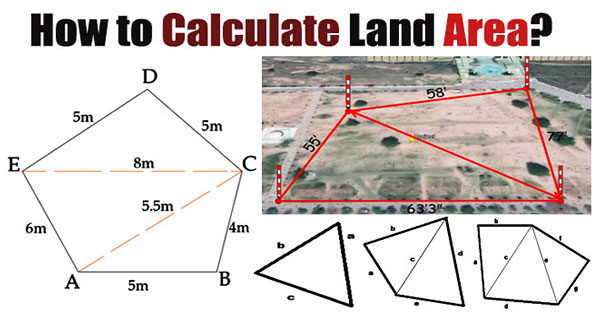### How to determine the area of simple geometric shapesThis civil engineering article mainly focuses on the following topics:-

How to find out the area of simple geometric shapes?

How to find out the area of irregular shapes or property?

Misconception of engineers and common patwaris.

Measurement of the area by manually and varying it with the use of software for accuracy.

The following formulas are used to determine the area of different geometric shapes:-

Area of square

(Side)2

Or a x a = a2

Here a denotes side of square.

Area of rectangle

Or a x b

Area of right triangle = ½ (length x breadth)

Or

½ (area of rectangle)

Area of scalene triangle

It is calculated with heron's formula i.e. √s(s-a) (s-b) (s-c)

S = a+b+c/2

Here, a/b/c denotes the sides of the triangle.

Heron formula stands for a method for measuring the area of a triangle when the lengths of all three sides are known.

Area of Equilateral triangle

½ (Side x Height)

Or

Area of equilateral triangle

= 0.433 (Side)2

Area of circle (taken as A)

A = πR2

Or

Area of circle = A = πD2 / 4

Here, D = diameter of circle

R denotes radius of circle

Area of regular polygon

½ (perimeter) (perpendicular)

Here, Perimeter is equivalent to sum of sides.

Perpendicular is equivalent to distance b/w edge center and the center of polygon.

To determine the area of any irregular shape property, it is necessary to convert or split the property into various triangles and then measure the area of each triangle individually and then add them jointly to obtain the area of entire property.

To start with, just select the boundary of the property and identify the corners of the boundary by using ranging rods or arrows as well as P supports or sticks. Once all the corners are identified, then calculate all the edges of the property. After the calculation is completed for edges, take the measurements of the diagonals to obtain the triangles. If the diagonals are not selected, the exact area of the property can't be measured. After that level every triangle with numbers like 1 and 2. Then start manual calculation and verify it with software.

The following two formulas are used to determine the complete area of the shape or property. Among these formulas, one is correct and another is improper.

Improper area calculation

Area of the entire shape or property:-

Area = (A+C) /2 + (B+D) /2

Under this formula, get an average of the opposite sides and multiply it by the average of the other two sides to obtain the entire area of the specified shape.

Proper area calculation

Total area = Area of triangle 1 + area of triangle 2

Total area = area 1 + area 2

The area of triangle 1 will be calculated with Heron's formula as follow:-

Area1 = √s(s-a) (s-b) (s-c)

S = a+b+c /2